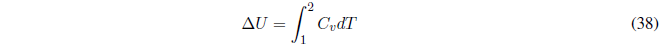In an adiabatic process, the walls of the system prevent heat exchange, therefore, $dq=0\;\;\rightarrow dU=dw$.

The internal energy variation can be calculated with:if $C_v$ remains constant,The enthalpy change is calculated with:Remember that in adiabatic processes the equations must be used:where $\gamma=\frac{C_p}{C_v}$